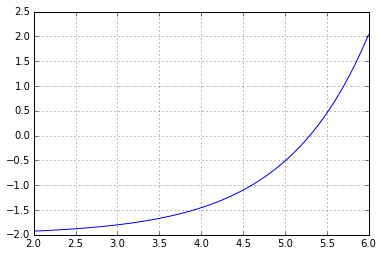# Bisection Method

In :
import numpy as np
import matplotlib.pyplot as pt

In :
a = 2
b = 6

x = np.linspace(a, b)

def f(x):
return 1e-2 * np.exp(x) - 2

pt.grid()
pt.plot(x, f(x))

Out:
[<matplotlib.lines.Line2D at 0x7f429c3f2438>]Write code for the bisection method and run it in-place many times: (Ctrl-Enter)

In :
m = (a+b)/2

if np.sign(f(a)) == np.sign(f(m)):
a = m
else:
b = m

print(a, b)

5.298316955566406 5.2983174324035645